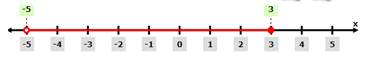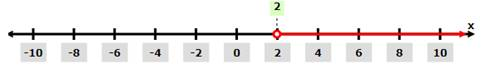# The graph of intervals ( − 5 , 3 ] and ( 2 , ∞ ) on the real number line.### Precalculus: Mathematics for Calcu...

6th Edition
Stewart + 5 others
Publisher: Cengage Learning
ISBN: 9780840068071### Precalculus: Mathematics for Calcu...

6th Edition
Stewart + 5 others
Publisher: Cengage Learning
ISBN: 9780840068071

#### Solutions

Chapter 1, Problem 1T

(a)

To determine

## To evaluate: The graph of intervals (−5,3] and (2,∞) on the real number line.

Expert Solution

The graphs of intervals are shown in Figure (1) and Figure (2).

### Explanation of Solution

Given:

The intervals are (5,3] and (2,) .

Calculation:

Section1:

The set description of interval (5,3] is {x|5<x3} because the set of all real number in between 5 and 3 called as intervals included the endpoint 3.

The intervals shows open interval from endpoint 5 and close interval from endpoint 3 that why the endpoint 5 does not include.

The graph of interval (5,3] is shown below,Figure (1)

Figure (1) shows the open interval from 5 and close interval from 3 on the real line number which represents the interval (5,3] .

Section2:

The set description of interval (2,) is {x|2<x} because the interval includes all real number after x=2 and does not include endpoints.

The graph of interval (2,) is shown below,Figure (2)

Figure (2) shows the graph of interval (2,) and does include endpoint 2 because it is a open interval.

(b)

To determine

### To express: The given inequalities in interval notation.

Expert Solution

The interval notation of given inequalities are (,3] and [1,4) .

### Explanation of Solution

Given:

The given inequalities are x3 and 1x<4 .

Calculation:

The inequality x3 shows that close interval of all set of real numbers before x=3 and includes the end point of this close interval so the interval notation of inequality x3 is (,3] .

The inequality 1x<4 shows the set of all real numbers from close interval 1 to open interval 4 and this interval includes endpoint 1 . so the interval for this inequality is [1,4)

Thus, the interval notation of given inequalities are (,3] and [1,4) .

(c)

To determine

### To find: The distance between −7 and 9 on the real number line.

Expert Solution

The distance is 16 in between 7 and 9.

### Explanation of Solution

Given:

The points is 7 and 9 on the real number line.

Calculation:

The formula of distance of points a and b is,

d(a,b)=|ba|

Where,

• d is distance in between numbers a and b.

Substitute 9 for b and 7 for a in the above formula to get the value of distance,

d(a,b)=|9(7)|=|9+7|=16

The distance between the points 7 and 9 on the real line number is shown below,Figure (3)

Figure (3) shows the distance between points 7 and 9.

Thus, the distance is 16 in between 7 and 9.

### Have a homework question?

Subscribe to bartleby learn! Ask subject matter experts 30 homework questions each month. Plus, you’ll have access to millions of step-by-step textbook answers!